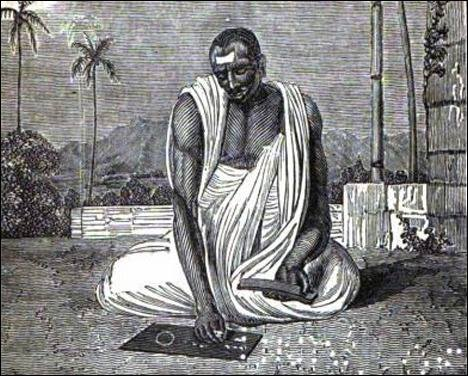Posted on: 4 January 2015

Digital Rare Book:
Brahmagupta's BRAHMA-SPHUTA SIDDHANTA
Edited by Acharyavara Ram Swarup Sharma
Published by Indian Institute of Astronomical and Sanskrit Research, New Delhi - 1965
In Four Volumes

In 628 ca. Indian mathematician and astronomer of Bhinmal, a town in the Jalore District of Rajasthan, India, Brahmagupta wrote Brahmasphutasiddhanta.

"It contains some remarkably advanced ideas, including a good understanding of the mathematical role of zero, rules for manipulating both negative and positive, a method for computing aquare roots, methods of solving linear and some quadratic equations, and rules for summing series, Brahamgupta's identity, and the Brahmagupta's theorem."

By this time a base 10 numeral system with nine symbols was widely used in India, and the concept of zero (represented by a dot) was known.

The book was written completely in verse and does not contain any kind of mathematical notation. Nevertheless, it contained the first clear description of the quadratic formula (the solution of the quadratic equation).

VOLUME 1:

VOLUME 2:
http://bit.ly/1IbLKr4

VOLUME 3:
http://bit.ly/17aSV7I

VOLUME 4:
http://bit.ly/1tITUX0

VOLUME 1:
http://bit.ly/1xojoJ5

VOLUME 2:
http://bit.ly/1xtol2S

VOLUME 3:
http://bit.ly/1xHuwPM

VOLUME 4:
http://bit.ly/1xHuLu1

Image:
Brahmagupta (Sanskrit: ब्रह्मगुप्त; About this sound listen (help·info)) (598–c.670 CE) was an Indian mathematician and astronomer who wrote two works on Mathematics and Astronomy: the Brāhmasphuṭasiddhānta (Extensive Treatise of Brahma) (628), a theoretical treatise, and the Khaṇḍakhādyaka, a more practical text. There are reasons to believe that Brahmagupta originated from Bhinmal.

Brahmagupta was the first to give rules to compute with zero. The texts composed by Brahmagupta were composed in elliptic verse, as was common practice in Indian mathematics, and consequently have a poetic ring to them. As no proofs are given, it is not known how Brahmagupta's mathematics was derived.
- WikiBrhmasphuta-siddhanta is one of the first mathematical books to provide concrete ideas on positive numbers, negative numbers, and zero. He wrote the following rules: The sum of two positive quantities is positive The sum of two negative quantities is negative The sum of zero and a negative number is negative The sum of zero and a positive number is positive The sum of zero and zero is zero The sum of a positive and a negative is their difference; or, if they are equal, zero In subtraction, the less is to be taken from the greater, positive from positive In subtraction, the less is to be taken from the greater, negative from negative When the greater however, is subtracted from the less, the difference is reversed When positive is to be subtracted from negative, and negative from positive, they must be added together The product of a negative quantity and a positive quantity is negative The product of two negative quantities is positive The product of two positive quantities is positive Positive divided by positive or negative by negative is positive Positive divided by negative is negative. Negative divided by positive is negative A positive or negative number when divided by zero is a fraction with the zero as denominator Zero divided by a negative or positive number is either zero or is expressed as a fraction with zero as numerator and the finite quantity as denominator Zero divided by zero is zero The last of these rules is notable as the earliest attempt to define division by zero, even though it is not compatible with modern number theory (division by zero is undefined for a field). - WikiSlap this on that NASA Indian Scientist who has started a petition against us.Thank you Geeta, will forward this to astronomersSorry sir, thanks to DOT India, archive.org has been blocked; and one cannot enjoy your uploads.!!The method of astronomical multiplications in ancient India was done in this way: ( nowadays we are daily confronted with these digits)